Courses

# Constant Electric Field In Vacuum (Part - 2) - Electrodynamics, Irodov JEE Notes | EduRev

## NEET : Constant Electric Field In Vacuum (Part - 2) - Electrodynamics, Irodov JEE Notes | EduRev

The document Constant Electric Field In Vacuum (Part - 2) - Electrodynamics, Irodov JEE Notes | EduRev is a part of the NEET Course Physics Class 12.
All you need of NEET at this link: NEET

Q. 18. Find the electric field strength vector at the centre of a ball of radius R with volume charge density p = ar, where a is a constant vector, and r is a radius vector drawn from the ball's centre.

Solution.18. Let us consider an elemental spherical shell of thickness dr. Thus surface charge density of the shell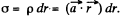Thus using the solution of Q.16, field strength due to this sperical shell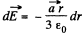Hence the sought field strength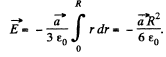Q. 19. A very long uniformly charged thread oriented along the axis of a circle of radius R rests on its centre with one of the ends. The charge of the thread per unit length is equal to λ. Find the flux of the vector E across the circle area.

Solution.19. From the solution of Q.14 field strength at a perpendicular distance r < R from its left end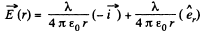Here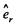is a unit vector along radial direction.

Let us consider an elemental surface,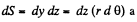figure. Thus flux of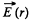over the element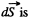given by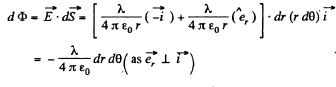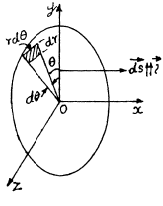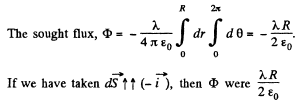Hence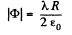Q. 20. Two point charges q and —q are separated by the distance 21 (Fig. 3.3). Find the flux of the electric field strength vector across a circle of radius R.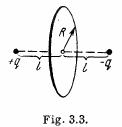Solution. 20. Let us consider an elemental surface area as shown in the figure. Then flux of the vector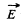through the elemental area,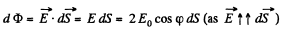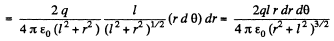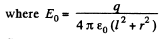is magnitude of field strength due to any point charge at the point of location of considered elemental area.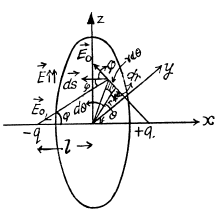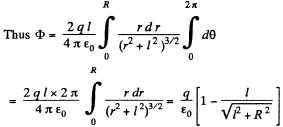It can also be solved by considering a ring element or by using solid angle.

Q. 21. A ball of radius R is uniformly charged with the volume density p. Find the flux of the electric field strength vector across the ball's section formed by the plane located at a distance r0 < R from the centre of the ball.

Solution. 21. Let us consider a ring element of radius x and thickness dx, as shown in the figure. Now, flux over the considered element,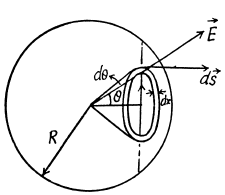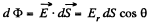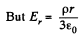from Gauss's theorem,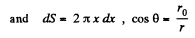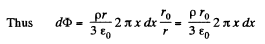Hence sought flux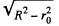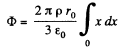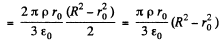Q. 22. Each of the two long parallel threads carries a uniform charge per unit length. The threads are separated by a distance l. Find the maximum magnitude of the electric field strength in the symmetry plane of this system located between the threads.

Solution. 22. The field at P due to the threads at A and B are both of magnitude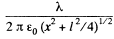and directed along AP and BP. The resultant is along OP with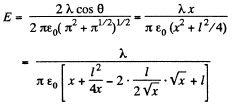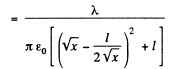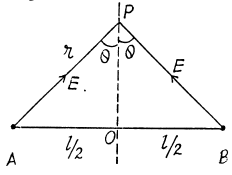This is maximum when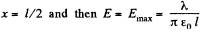Q. 23. An infinitely long cylindrical surface of circular crosssection is uniformly charged lengthwise with the surface density σ = σ0 cos φ, where φ is the polar angle of the cylindrical coordinate system whose z axis coincides with the axis of the given surface. Find the magnitude and direction of the electric field strength vector on the z axis.

Solution. 23. Take a section of the cylinder perpendicular to its axis through the point where the electric field is to be calculated. (All points on the axis are equivalent.) Consider an element S with azimuthal angle φ. The length of the element is R,dφ, R being the radius o f cross section of the cylinder. The element itself is a section of an infinite strip. The electric field at O due to this strip is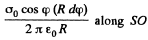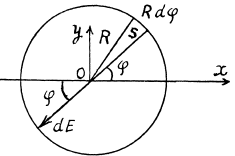This can be resolved into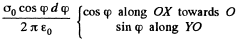On integration the component along YO vanishes. What remains is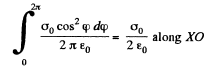Q. 24. The electric field strength depends only on the x and y coordinates according to the law E = a (xi + yj)/(x2 + y2), where a is a constant, i and j are the unit vectors of the x and y axes. Find the flux of the vector E through a sphere of radius R with its centre at the origin of coordinates.

Solution. 24. Since the field is axisymmetric (as the field of a uniformly charged filament ), we conclude that the flux through the sphere of radius R is equal to the flux through the lateral surface of a cylinder having the same radius and the height 2R, as arranged in the figure.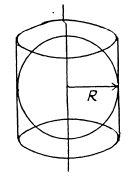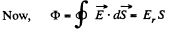But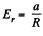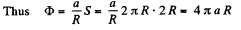Q. 25. A ball of` radius R carries a positive charge whose volume density depends only on a separation r from the ball's centre as P = p0 (1 — r/R), where P0 is a constant. Assuming the permittivities of the ball and the environment to be equal to unity, find:
(a) the magnitude of the electric field strength as a function of the distance r both inside and outside the ball;
(b) the maximum intensity Emax and the corresponding distance rm.

Solution. 25. (a) Let us consider a sphere of radius r < R then charge, inclosed by the considered sphere,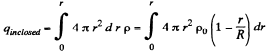(1)

Now, applying Gauss’ theorem,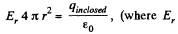is the projection o f electric field along the radial lin e.)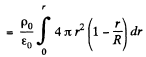or,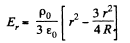And for a point, outside the sphere r > R.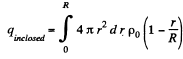(as there is no charge outside the ball)

Again from Gauss’ theorem,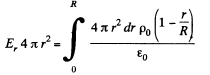or,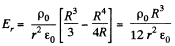(b) As‘magnitude of electric field decreases with increasing r for r > R, field will be maximum for r < R. Now, for Er to be maximum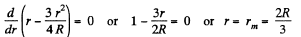Hence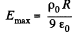Q. 26. A system consists of a ball of radius R carrying a spherically symmetric charge and the surrounding space filled with a charge of volume density p = α/r, where α is a constant, r is the distance from the centre of the ball. Find the ball's charge at which the magnitude of the electric field strength vector is independent of r outside the ball. How high is this strength? The permittivities of the ball and the surrounding space are assumed to be equal to unity.

Solution. 26. Let the charge carried by the sphere be q, then using Gauss’ theorem for a spherical surface having radius r > R, we can write.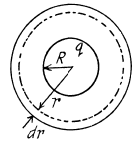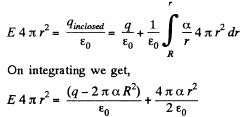The intensity E does not depend on r when the experession in the parentheses is equal to zero. Hence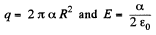Q. 27. A space is filled up with a charge with volume density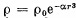where P0 and α are positive constants, r is the distance from the centre of this system. Find the magnitude of the electric field strength vector as a function of r. Investigate the obtained expression for the small and large values of r, i.e. at αr3 ≪1  and αr3 ≫ 1.

Solution. 27. Let us consider a spherical layer of radius r and thickness dr, having its centre coinciding with the centre of the system. Then using Gauss’ theorem for this surface,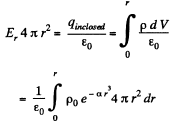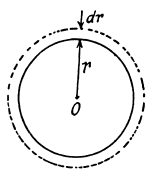After integration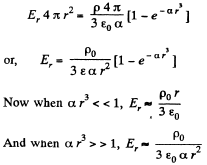Q. 28. Inside a ball charged uniformly with volume density p there is a spherical cavity. The centre of the cavity is displaced with respect to the centre of the ball by a distance a. Find the field strength E inside the cavity, assuming the permittivity equal to unity.

Solution. 28. Using Gauss theorem we can easily show that the electric field strength within a uniformly charged sphere is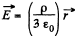The cavity, in our problem, may be considered as the superposition of two balls, one with the charge density p and the other with - p.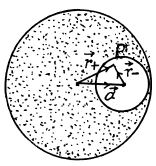Let P be a point inside the cavity such that its position vector with respect to the centre of cavity be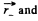with respect to the centre of the ball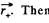Then from the principle of superposition, field inside the cavity, at an arbitrary point P ,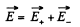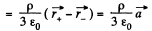Note : Obtained expression for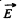shows that it is valid regardless of the ratio between the radii of the sphere and the distance between their centres.

Q. 29. Inside an infinitely long circular cylinder charged uniformly with volume density p there is a circular cylindrical cavity. The distance between the axes of the cylinder and the cavity is equal to a. Find the electric field strength E inside the cavity. The permittivity is assumed to be equal to unity.

Solution. 29. Let us consider a cylinderical Gaussian surface of radius r and height h inside an infinitely long charged cylinder with charge density p. Now from Gauss theorem :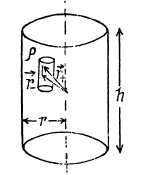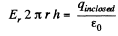(where Er is the field inside the cylinder at a distance r from its axis.)

or,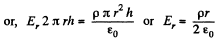Now, using the method of Q.28 field at a point P, inside the cavity, is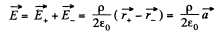Q. 30. There are two thin wire rings, each of radius R, whose axes coincide. The charges of the rings are q and —q. Find the potential difference between the centres of the rings separated by a distance a.

Solution. 30. The arrangement of the rings are as shown in the figure. Now, potential at the point 1, φ1 = potential at 1 due to the ring 1 + potential at 1 due to the ring 2.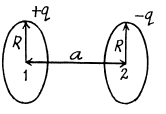Hence, the sought potential difference,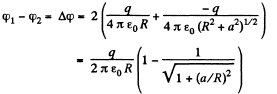Q. 31. There is an infinitely long straight thread carrying a charge with linear density λ, = 0.40 μC/m. Calculate the potential difference between points 1 and 2 if point 2 is removed η = 2.0 times farther from the thread than point I.

Solution. 31. We know from Gauss theorem that the electric field due to an infinietly long straight wire, at a perpendicular distance r from it equals,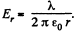So, the work done is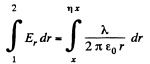(where x is perpendicular distance from the thread by which point 1 is removed from it.)

Hence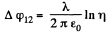Q. 32. Find the electric field potential and strength at the centre of a hemisphere of radius R charged uniformly with the surface density σ.

Solution. 32. Let us consider a ring element as shown in the figure. Then the charge, carried by the element,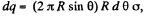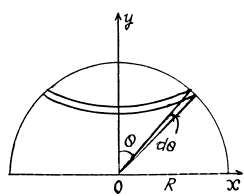Hence, the potential due to the considered element at the centre of the hemisphere,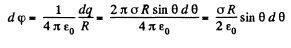So potential due to the whole hemisphere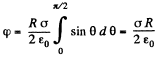Now from the symmetry of the problem, net electric field of the hemisphere is directed towards the negative y - axis. We have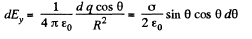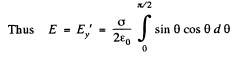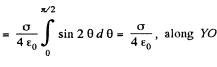Q. 33. A very thin round plate of radius R carrying a uniform surface charge density a is located in vacuum. Find the electric field potential and strength along the plate's axis as a function of a distance l from its centre. Investigate the obtained expression at l → 0 and l ≫ R.

Solution. 33. Let us consider an elementary ring of thickness dy and radius y as shown in the figure. Then potential at a point P, at distance l from the centre of the disc, is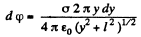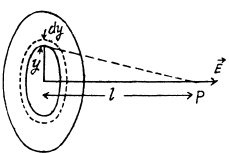Hence potential due to the whole disc,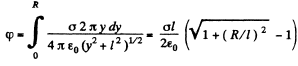From symmetry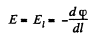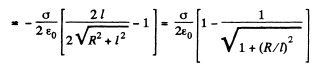Q. 34. Find the potential φ at the edge of a thin disc of radius R carrying the uniformly distributed charge with surface density σ.

Solution. 34. By definition, the potential in the case of a surface charge distribution is defined by integral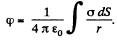In order to simplify integration, we shall choose the area element dS  in the form of a part of the ring of radius r and width dr in (Fig.). Then dS - 2θ r dr, r = 2R cos θ and dr = - 2R sin θ d θ. After substituting these expressions into integral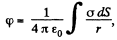we obtain the expression for cp at the point O: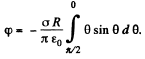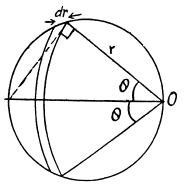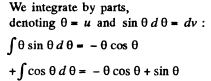which gives -1 after substituting the limits of integration. As a result, we obtain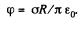Offer running on EduRev: Apply code STAYHOME200 to get INR 200 off on our premium plan EduRev Infinity!

## Physics Class 12

132 videos|306 docs|130 tests

,

,

,

,

,

,

,

,

,

,

,

,

,

,

,

,

,

,

,

,

,

,

,

,

;